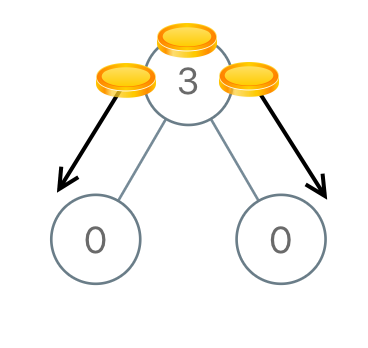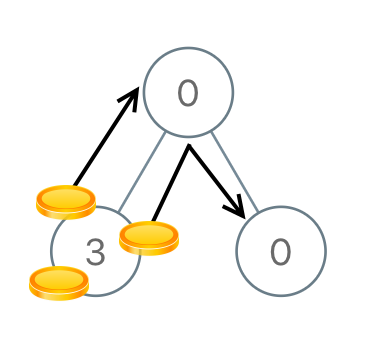# Distribute Coins in Binary Tree

## 1: U-nderstand

Understand what the interviewer is asking for by using test cases and questions about the problem.

• Established a set (2-3) of test cases to verify their own solution later.
• Established a set (1-2) of edge cases to verify their solution handles complexities.
• Have fully understood the problem and have no clarifying questions.
• Have you verified any Time/Space Constraints for this problem?
• Can the input tree be Null?
• No, there will be at least one node.
• Will the total number of coins always be the total number of nodes?
• Yes, there will be alway be 1 coin for nodes.
• What is the time and space constraints for this problem?
• `O(N)` time and `O(1)` space if we do not count the recursion stack.
``````HAPPY CASE
Input: root = [3,0,0]
Output: 2
Explanation: From the root of the tree, we move one coin to its left child, and one coin to its right child.````````````Input: root = [0,3,0]
Output: 3
Explanation: From the left child of the root, we move two coins to the root [taking two moves]. Then, we move one coin from the root of the tree to the right child.````````````EDGE CASE
Input: root = ,
Output: 0
Explanation: Since the tree is has only one coin and one node, no moves are made.``````

## 2: M-atch

Match what this problem looks like to known categories of problems, e.g. Linked List or Dynamic Programming, and strategies or patterns in those categories.

If you are dealing with Binary Trees some common techniques you can employ to help you solve the problem:

• Think about appropriate Tree Traversal: Pre-Order, In-Order, Post-Order, Level-Order
• Choosing a specific tree traversal that follows a general root-to-leaf path should help us move from leaf-to-root during the recursive return call.
• Store nodes within a HashMap to refer to later
• We don’t have a specific way of referring to previous nodes in a path that could be used in a HashMap. So, a HashMap would not help us as much in this context.
• Using Binary Search to find an element
• We are not working with a Binary Search Tree.
• Applying a level-order traversal with a queue
• Using this approach may complicate our code

## 3: P-lan

Plan the solution with appropriate visualizations and pseudocode.

General Idea: We will determine the number of moves needed at each node and sum those moves to get the total number of moves. The number of moves per node is the absolute value of both the left child and right child, because we need to remove or add that particular number of coins from this node to satisfy the condition of a single coin per node. The balance that needs to be moved from the left children or right children is the result of all the coins from the recursive call to left child and right child, itself, and -1 for the one coin that needs to stay.

``````1. We will determine the number of moves needed by looking at the balance of coins at each node that needs to be moved.
2. Create helper method to return the balance at each node. We will need to move this balance, hence we will record the balance move through this node.
a. Basecase: The balance (excess/needed) is 0 for nodes that don't exist.
b. Recursively check the number of coins needed to be moved per node starting from the leaves of tree as we need this for each node's total balance
c. Set total balance at this node is calculated with this formula. All the coins from left child, right child, itself, and the one coin it needs.
d. While calculating the balance of each node in this recursion, we will calculate the number of moves needed to make this balance from left and right child
e. Send the node's balance up to parent
3. Create variable to hold total number of moves.
4. Call helper method to populate total number of moves.

⚠️ Common Mistakes

• Choosing the wrong traversal type

• Try to walk through the problem by hand and see the order in which you are processing the nodes. This will clue you into the type of traversal necessary
• Suppose you attempted Level-order traversal, how would you determine the number of coins needed to be moved?
• We need a helper method to retain the total number of moves during the recursive calls.

## 4: I-mplement

Implement the code to solve the algorithm.

``````# Definition for a binary tree node.
# class TreeNode:
#     def __init__(self, val=0, left=None, right=None):
#         self.val = val
#         self.left = left
#         self.right = right
class Solution:
# We will determine the number of moves needed by looking at the balance of coins at each node that needs to be moved.
def distributeCoins(self, root: Optional[TreeNode]) -> int:
# Create helper method to return the balance at each node. We will need to move this balance, hence we will record the balance move through this node.
def helper(node):
nonlocal total_num_moves

# The balance (excess/needed) is 0 for nodes that don't exist
if not node:
return 0

# Recursively check the number of coins needed to be moved per node starting from the leaves of tree as we need this for each node's total balance
left_balance = helper(node.left)
right_balance = helper(node.right)

# Set total balance at this node is calculated with this formula. All the coins from left child, right child, itself, and the one coin it needs.
curr_node_balance = left_balance + right_balance + node.val - 1

# While calculating the balance of each node in this recursion, we will calculate the number of moves needed to make this balance from left and right child
n_moves_needed_to_balance_curr_node = abs(left_balance) + abs(right_balance)
total_num_moves+=n_moves_needed_to_balance_curr_node

# Send the node's balance up to parent
return curr_node_balance

# Create variable to hold total number of moves
total_num_moves = 0

# Call helper method to populate total number of moves
helper(root)

``````class Solution
{
// We will determine the number of moves needed by looking at the balance of coins at each node that needs to be moved.

// Create variable to hold total number of moves
int moves= 0;
public int distributeCoins(TreeNode root)
{
// Call helper method to populate total number of moves
helper(root);

return moves;
}

// Create helper method to return the balance at each node. We will need to move this balance, hence we will record the balance move through this node.
public int helper(TreeNode root)
{//Postorder traversal, we know about the child situation first and then the parent
// The balance (excess/needed) is 0 for nodes that don't exist
if(root == null)
return 0;
// Recursively check the number of coins needed to be moved per node starting from the leaves of tree as we need this for each node's total balance
int coinsLeft= helper(root.left);
int coinsRight= helper(root.right);

// Set total balance at this node is calculated with this formula. All the coins from left child, right child, itself, and the one coin it needs.
int coins= coinsLeft + coinsRight;

// While calculating the balance of each node in this recursion, we will calculate the number of moves needed to make this balance from left and right child
//root node situation
if(root.val == 0) //root node cannot contribute, but needs coin from its parent
coins-= 1;//current node need one coin from parent

else if(root.val == 1)//root node cannot contribute, at par
coins+= 0;

else //root node can contribute to its parent
coins+= root.val- 1;//excess coin are been transfered to the parent

moves+= Math.abs(coins);

// Send the node's balance up to parent
return coins;
}
}``````

## 5: R-eview

Review the code by running specific example(s) and recording values (watchlist) of your code's variables along the way.

• Trace through your code with an input to check for the expected output
• Catch possible edge cases and off-by-one errors

## 6: E-valuate

Evaluate the performance of your algorithm and state any strong/weak or future potential work.

Assume `N` represents the number nodes in tree

• Time Complexity: `O(N)` because we need to visit each node in binary tree.
• Space Complexity: `O(1)` if we do not count the recursion stack. The recursion stack will cost us `O(N)` if we have a linked list like tree. `O(logN)` in the average case.